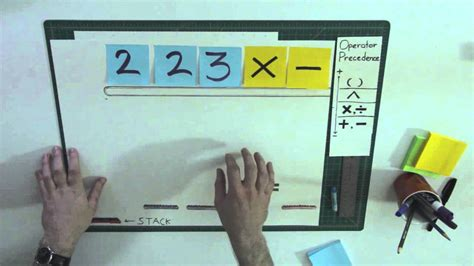The buttons on your postfix-notation calculator each come with a cost. You can push any operator (plus, minus, times, floored division, modulo, and copy) onto the stack for a cost of one. You can also push any integer (say, in a fixed range 1...9) onto the stack; the cost is the value of the integer itself. Find the smallest-cost program for producing any integer.A postfix calculator (https://www.youtube.com/watch?v=y_snKkv0gWc)For example, optimal programs for the first few integers are 1, 2, 3, 2@+, 5, 3@+, 13@++, 2@@**, 3@* (Here @ denotes the operator which puts a copy of the top element of the stack onto the stack.)

You can find short programs using branch-and-bound search to search the space of stack states. There are some optimizations:

• Using dynamic programming (Dijkstra's algorithm), you can cache the lowest-cost path to each state so that you never have to expand a state more than once.
• Second, you can use the size of the stack state as an admissible heuristic: if a stack has n items in it, it will take at least n-1 binary operators to collapse it into single number.

There's a nice theorem, too: For any integer, the lowest-cost program only ever needs the constants 1 through 5 (additional constants never offer additional savings). The program never costs more than the integer itself, and in fact for sufficiently large integers (over 34), it costs less than half of the integer. To prove the theorem, first show that every integer n has a (possibly nonoptimal) program which costs at most n and which uses the constants 1...5.

Proof: Check, by hand, that you can make the first 34 numbers with programs that don't cost more than the number and that use only the digits 1...5 (For any smaller set of digits, this part fails because 5 costs more than 5 to make.) For cases larger than 34, a stronger property emerges: the optimal 1...5 program for each integer costs less than half the integer itself, minus one (!). You can prove this by induction. Check cases 34 through 256 by hand. Then if the theorem is true for every integer between 34 and some power of two, it is true for every integer between that power of two and the next one. Indeed, any integer in that range can be written as the sum of two numbers larger than 34, and by the induction hypothesis, the program which generates those two numbers then adds them costs less than half the integer.

Next, suppose you have an optimal-cost program which uses any set of constants. By the previous result, you can replace each constant with a short script that uses only 1...5, and this substitution won't increase the program's cost. Hence the program-with-substitutions is still optimal, but only uses the digits 1...5, QED.